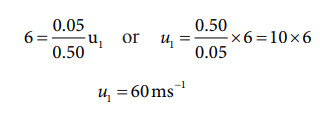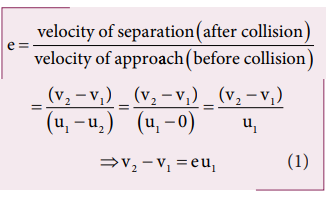Home | | Physics 11th std | Solved Example Problems for Collision

# Solved Example Problems for Collision

Physics : Work, Energy and Power : Collision

Solved Example Problems for collision

## Solved Example Problems for Elastic collisions in one dimension

### Example 4.20

A lighter particle moving with a speed of 10 m s-1 collides with an object of double its mass moving in the same direction with half its speed. Assume that the collision is a one dimensional elastic collision. What will be the speed of both particles after the collision?

### SolutionLet the mass of the fi rst body be m which  moves with an initial velocity, u1 = 10 m s-1.

Therefore, the mass of second body is 2m and its initial velocity is u2 = ½ u1 = ½(10ms-1)

Then, the fi nal velocities of the bodies can be calculated from the equation (4.53) and equation (4.54)As the two speeds v1and v2 are positive, they move in the same direction with the velocities, 3.33 m s−1 and 8.33 m s−1 respectively.

## Solved Example Problems for Perfect inelastic collision

### Example 4.21

A bullet of mass 50 g is fired from below into a suspended object of mass 450 g. The object rises through a height of 1.8 m with bullet remaining inside the object. Find the speed of the bullet. Take g = 10 ms-2.

### Solution

m1 = 50 g = 0.05 kg; m2 = 450 g = 0.45kgThe speed of the bullet is u1. The second body is at rest u2 = 0 . Let the common velocity of the bullet and the object after the bullet is embedded into the object is v.The combined velocity is the initial velocity for the vertical upward motion of the combined bullet and the object. From second equation of motion,Substituting this in the above equation, the value of u1 is## Solved Example Problems for Coefficient of restitution (e)

### Example 4.22

Show that the ratio of velocities of equal masses in an inelastic collision when one of the masses is stationary is

v1/v2 = 1-e/1+e

### SolutionFrom the law of conservation of linear momentum,Using the equation (2) for u1 in (1), we getStudy Material, Lecturing Notes, Assignment, Reference, Wiki description explanation, brief detail
11th Physics : UNIT 4 : Work, Energy and Power : Solved Example Problems for Collision |